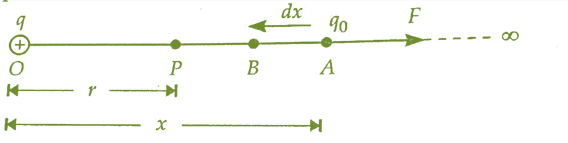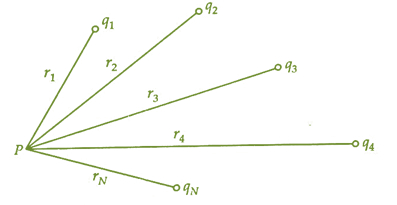# Electric Potential Point Charge

## Introduction

Most people are very doubtful about the terminology ‘Potential’. In our school time when we first time listened to this term, we learned that potential is somewhat related to the position. However, it means capacity or strength. The term electric potential represents the strength of electricity.

When we start a study for electricity, the first thing we have to face is a charge. The charge is the base of electronics. It is of two types; positive and negative. As we proceed with the charge, we come to know about the electric field, electric potential, potential energy, dipole, etc. Every single charge or a cluster of charges has its field of influence where we can experience the force of attraction and repulsion or develop potential at every position in that region.

## What is Electric Potential?

In simple language, we can say that the electric potential for a point in a region where the effective electric field is present, is a piece of work we have to do to change the location of a positive point charge of magnitude one coulomb from infinity position to the point which is considered by us at which the effect of the electric field is present which continues exerting electrostatic force.

Hence, electric potential can also be expressed as work done on a unit charge. Volt is the standard measurement unit of Electric potential. Hence, a one-volt electric potential point is the one joule of work that has to be done for bringing a point positive charge which is located at an infinite far position to a target point. The complete process is also under the impact of forces that comes into play because of the electric field.

$$\mathrm{V=\frac{W}{q}}$$

## Electric Potential due to a Point Charge

As we all know that every charge and bunch of multiple charges have their region of influence known as the electric field. In that field, we can feel some electrostatic forces. A point charge also creates an electric potential. In the below section, we are going to examine the potential due to point charge.

Electric Potential at a test charge due to A Point ChargeHere, a positive charge q is at point O. Now, we are going to calculate electric potential at a point P which is r distance away from O. As the definition represents that electric potential is equal to the piece of work that is done by us to bring a unit positive charge from the infinite position to the considered point P.

Now, we suppose that there is a test charge $\mathrm{q_{0}}$ at x distance far from O point at A.

Using the Coulomb Law, we can find out the force experienced by a test charge because of the q charge,

$$\mathrm{F=\frac{1}{4\pi \epsilon _{0}}.\frac{qq_{0}}{x^{2}}}$$

As the force F due to the electric field is working in a direction which is in the opposite direction of the movement of $\mathrm{q_{0}}$ charge from location A to location B so we have to do a small amount of work for moving a charge by dxdisplacement against the force exerted by charge.

Hence,

$$\mathrm{dW=\overrightarrow{F}.\overrightarrow{dx}=F.dxcos\:180^{\circ}}$$

$$\mathrm{dW=-F\:dx}$$

The dW is the small amount of work.

The total work needed in the movement of the test charge from any infinite position to point P can find out by integration

$$\mathrm{W=\int dW=-\int_{\infty }^{r}F\:dx}$$

$$\mathrm{W=-\int_{\infty }^{r}\frac{1}{4\pi \epsilon _{0}}.\frac{qq_{0}}{x^{2}}\:dx}$$

$$\mathrm{W=-\frac{qq_{0}}{4\pi \epsilon _{0}}\int_{\infty }^{r}x^{-2}\:dx}$$

$$\mathrm{W=-\frac{qq_{0}}{4\pi \epsilon _{0}}\left [ -\frac{1}{x} \right ]_{\infty }^{r}}$$

$$\mathrm{W=\frac{qq_{0}}{4\pi \epsilon _{0}}\left [ \frac{1}{r}-\frac{1}{\infty } \right ]}$$

$$\mathrm{W=\frac{1}{4\pi \epsilon _{0}}.\frac{qq_{0}}{r}}$$

Thus, from the above expression, the piece of work required to change the position of a test charge from the point which is at an infinite position to the point P is

$$\mathrm{W=\frac{1}{4\pi \epsilon _{0}}.\frac{qq_{0}}{r}}$$

Now, we know that Potential is

$$\mathrm{V=\frac{W}{q_{0}}}$$

Hence,

$$\mathrm{V=\frac{1}{4\pi \epsilon _{0}}.\frac{q}{r}}$$

## Electric Potential due to Multiple Charges

Here we are going to measure the electric potential at any arbitrary point due to a group of N charges. Let there are N point charges i.e $\mathrm{q_{1},q_{2},q_{3},q_{4},q_{5},q_{6},q_{7}.....q_{N}}$ are present in the system we considered. All the point charges are $\mathrm{r_{1},r_{2},r_{3},r_{4},r_{5},r_{6},r_{7}.....r_{N}}$ distance away from the considered point P.From the last section, we know that the electric potential on any arbitrary point P because of a point charge is

$$\mathrm{V=\frac{1}{4\pi \epsilon _{0}}.\frac{q}{r}}$$

For charge $\mathrm{q_{1}}$

$$\mathrm{V_{1}=\frac{1}{4\pi \epsilon _{0}}.\frac{q_{1}}{r_{1}}}$$

Same like this for other $\mathrm{q_{1},q_{2},q_{3},q_{4},q_{5},q_{6},q_{7}.....q_{N}}$, we get

$$\mathrm{V_{1}=\frac{1}{4\pi \epsilon _{0}}.\frac{q_{1}}{r_{1}},V_{2}=\frac{1}{4\pi \epsilon _{0}}.\frac{q_{2}}{r_{2}},V_{3}=\frac{1}{4\pi \epsilon _{0}}.\frac{q_{3}}{r_{3}}............V_{N}=\frac{1}{4\pi \epsilon _{0}}.\frac{q_{N}}{r_{N}}}$$

We also know that electric potential is not a vector quantity it is a scalar quantity, so for calculating the total electric potential at any point P by N charges will be the sum of the individual potential of every charge.

Thus,

$$\mathrm{V=V_{1},V_{2},V_{3},V_{4}+........+V_{N}}$$

$$\mathrm{V=\frac{1}{4\pi \epsilon _{0}}.\frac{q_{1}}{r_{1}}+\frac{1}{4\pi \epsilon _{0}}.\frac{q_{2}}{r_{2}}+\frac{1}{4\pi \epsilon _{0}}.\frac{q_{3}}{r_{3}}............+\frac{1}{4\pi \epsilon _{0}}.\frac{q_{N}}{r_{N}}}$$

$$\mathrm{V=\frac{1}{4\pi \epsilon _{0}}\left [ \frac{q_{1}}{r_{1}}+ \frac{q_{2}}{r_{2}}+\frac{q_{3}}{r_{3}}+......+\frac{q_{N}}{r_{N}}\right ]}$$

We can also write the above equation in the form of summation,

$$\mathrm{V=\frac{1}{4\pi \epsilon _{0}}\sum_{i=1}^{N}\frac{q_{i}}{r_{i}}}$$

This is the total electric potential at any arbitrary point which is here denoted by P in the system of N charges.

## Conclusion

In the above sections, we have studied the electric potential on any point by a single charge and by a group of charges. We notice that electric potential is because a charge is spherically symmetric. Also, an electric potential is a scalar quantity so for a group of charges we can calculate it by simple addition.

## FAQs

Q1. Is it possible at any point we find the electric potential is zero but the electric field at that point is not zero?

Ans. Yes, we can find such a condition. We know that the electric potential at any equatorial point that lies perpendicular to the line of axis of the dipole is zero but when we test the electric field at that point we feel some of the influence of the electric field.

Q2. If we move along the electric line of force, what will happen to electric potential?

Ans. In this situation, we find that the electric potential will decrease along the electric line of force.

Q3. What is the Standard Unit in terms of Base Unit for Electric Potential?

Ans. The Base unit form of the Standard unit of potential is

$$\mathrm{Potential=\frac{Work\:done}{charge}}$$

Hence,

$$\mathrm{\frac{ML^{2}T^{-2}}{AT}=ML^{2}T^{-3}A^{-1}}$$

Q4. Why electric potential inside a conductor is constant?

Ans. We know that the electric potential has a direct relation with the electric field. The electric field inside any conductor is zero, so the electric potential in a conductor is constant. We can justify it by this expression,

$$\mathrm{E=-\frac{dV}{dr}}$$

When, E = 0

$$\mathrm{\frac{dV}{dr}=0}$$

$$\mathrm{V= Constant}$$

Q5. What do you mean by Equipotential Surface?

Ans. An equipotential surface is a surface on which every point has equal potential throughout the complete surface.